Zen Pharaohs of Ultimate Love mathematics, music, science, wood....what else could you ask?Next: Properties of the Maximally Up: Recursive Windowing of Time Previous: Interpolation and Irregularly Spaced

# Maximally Flat Impulse Response

A very nice filter for windowing can be given by choosing an exponential decay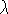, and settingand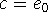. Then we will determine b so thatNow our choice of A by specifying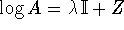looks more natural, and, noting that for k<d we have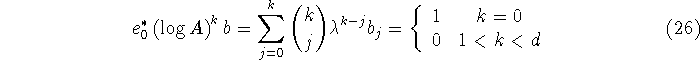and from the binomial theorem we have the solution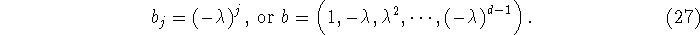We should also explain that since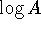has been chosen to be triangular, so is A, and the eigenvalues of, (in this case the repeated eigenvalue) is the logarithm of the repeated eigenvalue of A, namely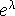. Since we want A to be a stable matrix, we have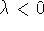.

Fri Jun 27 03:10:38 EDT 1997